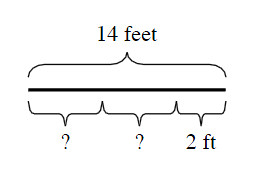### Home > MC2 > Chapter 1 > Lesson 1.2.2 > Problem1-61

1-61.

Kayla has a $14$-foot rope. She cut it into three parts. Two of the pieces are the same length and the third piece is $2$ feet long.

1. Copy Kayla's diagram above onto your paper.

1. How long were each of the two equal pieces?

How long is the entire rope?
What do the $3$ sections need to add up to?

If $? + ? + 2\text{ ft} = 14\text{ ft}....$
What are the values of the missing sections?

Remember, the two missing sections are equal to each other.